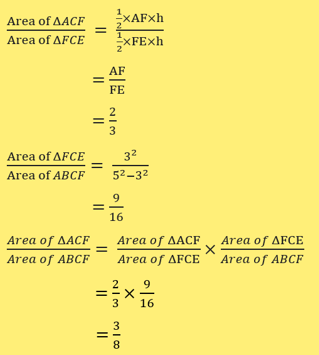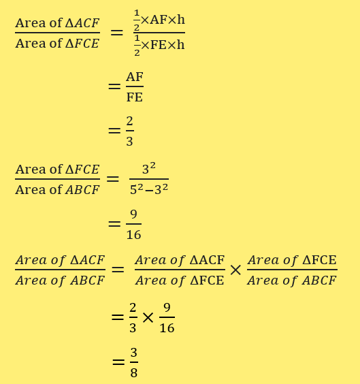### MATHEMATICS

Concept-Practice Framework

Our carefully curated notes and worksheets aim to break down concepts from textbooks into easily digestable bite-sized parts and arrange activities according to cognitive levels. Our curriculum is conceptually designed step-by-step such that our worksheets follow a progressive learning curve and the learning objectives of each topic closely. This enables students to grasp new concepts more easily and tackle challenging problems with confidence. Our step-by-step approach aids weaker students in building a strong foundation.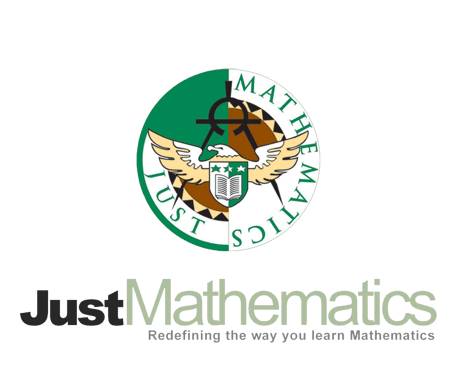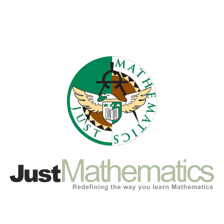JustEdu students get to try their hand at every single math concept ever tested in school. We will train our students to tackle higher order questions via Heuristics Training and Challenging Word Problems.

Our heuristics training challenges our students to a wide variety of questions that requires them to think out-of-the-box. They will be able to handle questions such as:

• Give a Representation (Diagram drawing, Making a list, Use equations)

• Make a Calculated Guess (Guess & check, Patterns, Making assumptions)

• Working out the Process (Act it out, Working backwards, Before and After)

• Changing the Problem (Restate the problem, Simplying the problem, Solve part of the problem)

Other popular word problems typically come from a selected range of topics like: Ratio, Rate, Fractions, Percentage, Volume, Speed. These problems sums specifically tests a student's spatial visualisation skills and application which requires practice and most importantly, tutorial guidance.

Our teachers are thoroughly trained and equipped with detailed worked solutions to guide students through each step of the problem.

Logical reasoning and multiple-concept questions involve real life applications and requires students to break down a word problem into multiple concept parts to activate relevant knowledge. Requiring the initial help of our tutors to help students visualise the problem, students will progressively activate and link their knowledge of various concepts, grasping a bird's eye view of the problem to work their way towards the solution.

### TEACHING APPROACH

When it comes to teaching methodology,

we look towards developing the 5 'E's in each of our students.

These are the five key tenets of our approach to Education

### Engage

Here, we engage our students and grab their attention before we start going through the topic by sharing with them:

• How the topic they are about to learn can be applied in real life

• History of Mathematics (Secondary)

• Discussions based on the students' initial understanding of the topic to make connections between past and present learning experiences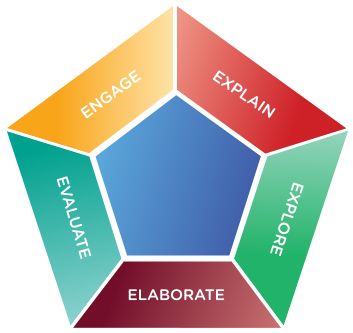### Lower Secondary

A contractor agrees to lay a road of length 3000 m in 20 days. He employs 80 men who work 8 hours a day. After 15 working days, he finds that only 2000 m of the road is completed. How many more men should he employ so as to finish the work on time if each man now works 10 hours a day?

### Show Solution

No. of hours worked in 15 days

= 8 x 5

= 120

Remaining no. of hours to work

= 5 x 10

= 50

Remaining length of road to lay

= 2000 - 1000

= 1000 m

Let the number of workers be x and the total number of hours be y. Since the number of workers is inversely proportional to the total number of hours,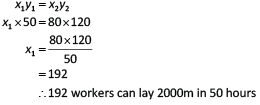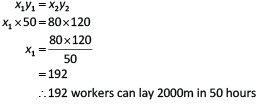Let the number of workers be x and the total length of road be I. Since the number of workers is directly proportional to the length of road,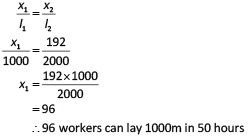Therefore, 96 - 80 = 16 more men required.

Gillian and Cadence packed 4000 presents for an orphanage.

The time Gillian took to pack 7 presents was similar to the time Cadence took to pack 9 presents.

They earned 45¢ for every present packed. An additional \$1.50 was earned for every 30 presents packed.

How much more did Cadence earn than Gillian?

Show Solution

4000 ÷ (7 + 9) = 250

250 x 7 = 1750

250 x 9 = 2250

1750 x 45¢ = \$787.50

1750 ÷ 30 = 58 R 10

\$787.50 + 58 x \$1.50 = \$874.50

2250 x 45¢ = \$1012.50

2250 ÷ 30 = 75

\$1012.50 + 75 x \$1.50 = \$1125

\$1125 - \$874.50 = \$250.50

Ans: \$250.50

### Upper Secondary

In a bridge structure, the mast above each pillar is 87 metres high.

The gradient at which the longest steel cable is connected to the centre of the bridge is such that the ratio of vertical distance : horizontal distance is 29 : 57.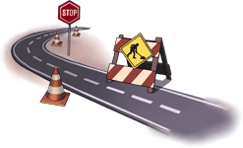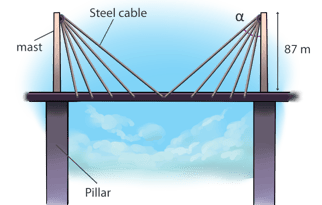a) Show that the angle, α, is 63.03° correct to 2 decimal places.

b) Calculate the length of 2 pieces of the longest steel cable attached from the top of the mast to the centre of the bridge.

Show Solution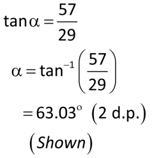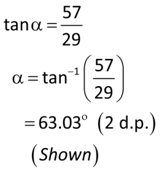a)

b)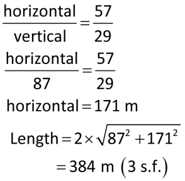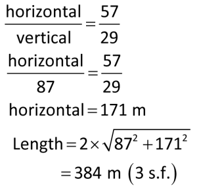In the diagram, ΔFCE is similar to ΔABE. Given that ΔABC has an area of 24 cm2, 3BC = 2CE and 4DE = DA,

a) find the area of ΔACE;

b) find the area of ΔFCE;

c) show that area ΔCDE = ½ area of ΔABC;

d) find the value of area of ΔACF

area of ABCF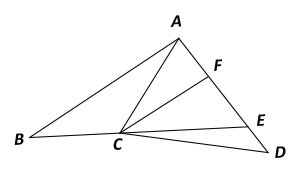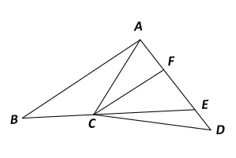Show Solution

a)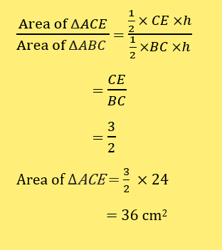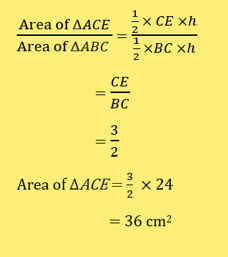b)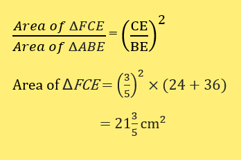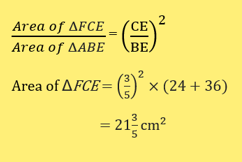c)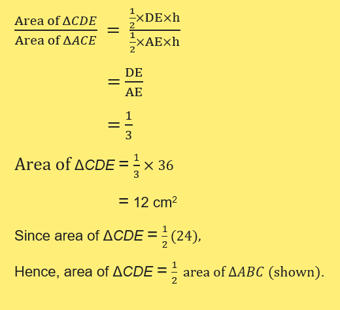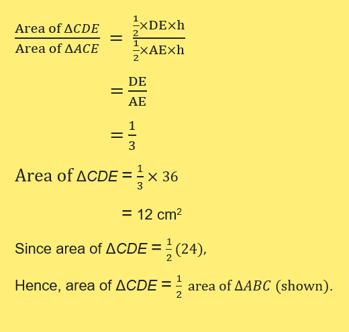d)